# Abbe-Method

Measuring method (named after Ernst Abbe) used to determine the focal length and the position of the principal planes of a lens singlet or a lens system (=objective) on the optical axis.

How to determine the focal length:
The position of the lens is fixed and the camera (or the screen ) is moved depending on the object position, that you get a focused image (in the image center). Different object positions result in different camera- or screen distancesHow to determine the focal length of an objective (= (= lens system)):
The Position of a lens (and the lens singlets in it) are fixed and an arbitrary Point O on the optical axis is marked as reference point, for example the center of the lens or the center of the first lens element).
Now we measure the distance x from the reference point to the object, the distance x’ to the image and the image size B.
You get a list of Magnifications,
and equations from refererence Point to objectand reference point to image:Where h und h’ are the distances from object side resp. image side principal planw to the reference point.

# Abbe-number

(also known as v-number)
a measure of the materials dispersion (=variation of refractive index with wavelength),
with high values of V indicating low dispersion (low chromatic aberration).

The value Vd is given bywhich defines the Abbe number with respect to the yellow Fraunhofer-Line d (or D3) helium line at 587.5618 nm wavelength.

It can also be defined using the green mercury E-line at 546.073 nm:where F’ and C’ are the blue and red cadmium lines at 480.0 nm and 643.8 nm, respectively.

# Abbe’s Invariant

In paraxial optics each single refracting surface satisfies the Abbe’s Invariant Q in the paraxial Area, that relates the front focal distance s of an axial object point with the back focal distance s’ of it’s conjugated point behind the surface# ABCD Matrix

ABCD Matrixesare used in paraxial optical design.

It’s only allowed to use ABCD Matrices in the paraxial range, withThe angles are measured in radians!

A beam is described by a distance r from the optical axis and a offset angle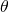from the optical axis
An ABCD Matrix that describes the optical element is formed
The ABCD Matrix is multiplied by the input vector
The result is an output vector that describes the output beam with a new distance from the optical axis and a new angle off the optical axisis a short for for the equation systemwithwith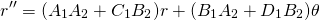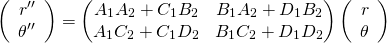Examples of ABCD matrices for simple optical elements :

Propagation in free space or in a medium of constant refractive index :Where d = reduced distance= thickness / refraction index
Propagation through a series i=1..k of planparallel media with constant refraction indices: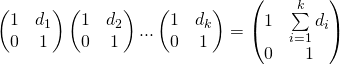Where d_i = reduced distance_i= thickness_i / refraction index_i

Refraction at a flat surface:Where:= initial refractive index= final refractive index
Refraction at a curved surface:Where:
R = radius of curvature,for a convex surface (centre of curvature after interface)= initial refractive index= final refractive index
Reflection vrom a flat mirror:Identity matrix
Reflection from a curved mirror:Where:effective radius of curvature in tangential plane (horizontal direction)effective radius of curvature in the sagittal plane (vertical direction)
Withfor convex mirrors (centre of curvature after interface)
Refraction at a thin lens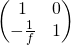Where:
f = focal length of the lens, wherefor convex/positive/converging lenses. Valid if if and only if the focal length is much bigger than the thickness of the lens
Refraction at a thick lensWhere:= refractive index outside of the lens.= refractive index of the lens itself (inside the lens).= Radius of curvature of First surface.= Radius of curvature of Second surface.
t = center thickness of lens.

# aberration

deviation from perfection of an optical system.

Even a perfectly produced optical system has aberrations. Production does produce additional aberrations.